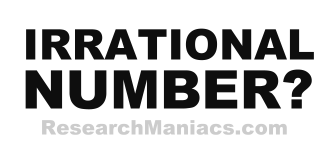Is 30 an Irrational Number?The number 30 is an irrational number if 30 canNOT be expressed as a ratio, as in irRATIOnal.

A quotient is the result you get when you divide one number by another number. For 30 to be an irrational number, the quotient of two integers canNOT equal 30.

In other words, for 30 to be an irrational number, 30 canNOT be expressed as a ratio where both the numerator and denominator are integers (whole numbers).

The number 30 can be expressed as 30/1 where 30 is the numerator and 1 is denominator.

Thus, the answer to the question "Is 30 an irrational number?" is NO.

Irrational Number?
Enter another number to see if it is irrational:

Is 31 an Irrational Number?
See information about the next number on our list.